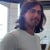# SAT/SMT Solvers

`      ∀x (f(x) = x * 42) ∧ (a ≥ b) ∧ (b ≥ c) ∧ (f(a) ≥ f(c))`
• a = 0, b = 0, c = 0
• a = 1, b = 1, c = 1
• a = 2, b = 1, c = 0
• a = 300, b = 200, c = 100

# Solidity Verification

`pragma experimental SMTChecker;contract C{    uint c;    function f(uint x) public pure returns (uint) {        return x * 42;    }    function g(uint a, uint b) public {        require(a >= b);        require(b >= c);        assert(f(a) >= f(c));    }}`
`      ∀x (f(x) = x * 42) ∧ (a ≥ b) ∧ (b ≥ c) ∧ (f(a) < f(c))`
• If the SMT solver answers that the formula is Satisfiable, it means that there are values for the program variables that satisfy the negation of the assertion, that is, the assertion can be violated and there is a bug. In that case the values are a counterexample that can be reported back to the developer.
• If the formula is Unsatisfiable, there is no program behavior that can break the assertion, therefore the assertion is correct.
`Warning: Assertion violation happens here  assert(f(a) == f(c));  ^------------------^  for:  a = 1  b = 1  c = 0`

# False Positives

`pragma experimental SMTChecker;contract C{    uint sum;    uint count;    function () external payable {        require(msg.value > 0);        require(sum + msg.value <= 1000000);        sum = sum + msg.value;        count = count + 1;    }    function average() public view returns (uint avg) {        require(count > 0);        avg = sum / count;        assert(avg > 0);        return avg;    }}`
`example.sol:19:3: Warning: Assertion violation happens here  assert(avg > 0);  ^-------------^  for:  avg = 0  count = 1  sum = 0`
• `msg.value` is an unsigned integer and the fallback function guarantees that it is least 1;
• `sum` is incremented by `msg.value` in the fallback function, where `count` is incremented by 1;
• from the points above we know that the invariant `sum >= count` holds in the beginning/end of every function.
`pragma experimental SMTChecker;contract C{    uint sum;    uint count;    function () external payable {        require(msg.value > 0);        require(sum + msg.value <= 1000000);        sum = sum + msg.value;        count = count + 1;    }    function average() public view returns (uint avg) {        require(count > 0);        require(sum >= count);        avg = sum / count;        assert(avg > 0);        return avg;    }}`

# References

## More from Leonardo Alt

Building Solidity and Formal Verification tools.

Love podcasts or audiobooks? Learn on the go with our new app.

## Windows For Power Users## Creating the Awesome Oscillator in Python## Everything is object?! with Python3🐍## Dynamic VS Static librarys & how use them:## Databases: Explaining Data Normalization, Data Anomalies and DBMS Keys## Leonardo Alt

Building Solidity and Formal Verification tools.

## NFT (ERC721) with Physical Asset Delivery and Secondary Royalties (ERC2981)## Yul vs Solidity Contract Comparison## Smart contracts: neither smart, nor contracts